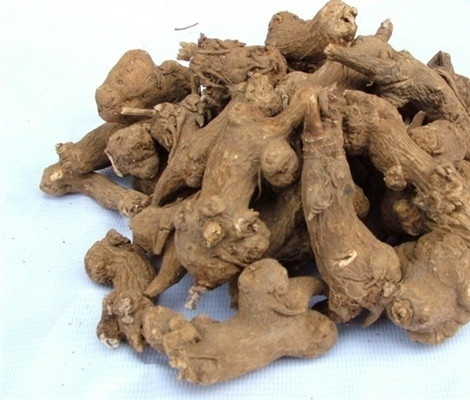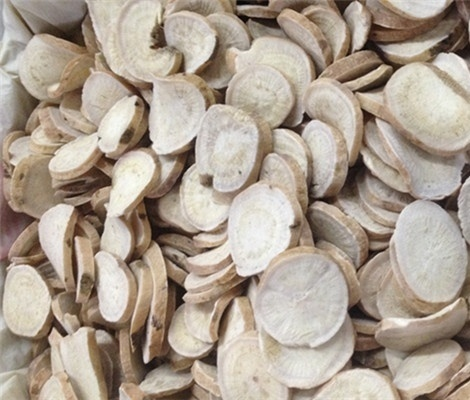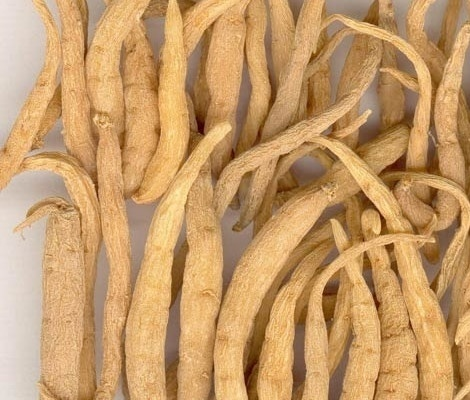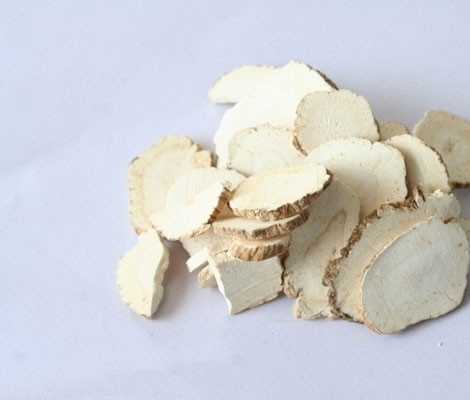|

# 南方药材种植什么比较好?

我们都知道，中医在我们国家的历史是非常的悠久的，当然了，中医文化也为我们流传下来了许许多多的药材处方，并且很多典籍之中，也为我们记载了许许多多的珍贵的药材。不过呢，不同的中药材，当然也有不同的生长习性，所以，许多药材的产地的差异，也是跟它们的生长习性有着非常大的关系的。接下来呢，小编就来为大家介绍一下南方药材种植哪些比较好。

(一)白芍

白芍是我国传统的名花之一，与牡丹合称为“花中二绝”是装点园林,美化环境的主要花卉。其根干燥为白芍，味苦、酸、性微寒，归肝、肺、脾经，具有平肝止痛、养血调经、敛阴止汗之功能，用于头脑眩晕，胁痛、腹痛，肌肉挛痛、月经不调、胎动不安、自汗、盗汗等症。

芍药适宜温暖湿润气候条件，具有喜光、喜温、喜肥和一定的耐寒特性。在年均温14.5℃，7月均温27.8℃，极端最高温42.1℃的条件下生长良好。

芍药具有很高的观赏和药用价值，其种植效益显著。市场潜力很大，是一项新兴产业。种植采用芽头，亩用种苗2800-3000株，前三年在白芍的行距内可以套作农作物和经济作物。况且不影响农作物产量，也可在树下套作。最佳种植时间9月15日-11月20日。

(二)牡丹

牡丹系毛茛科落叶小灌木，为常用中药，以根皮人药。牡丹适应性强，产量高，质量好，每667米2可收干丹皮500公斤。牡丹种植分种子直播和育苗移栽两种。种子直播时间是9月15日-10月底。亩用种子50-60公斤，三年收获;种苗移栽，亩用种苗6000株，生长期4-5年，前三年可以套作农作物，也可在树下种植，产量低于大田种植。(三)白芷

白芷又名川白芷、香白芷等，为伞形科当归属植物，白芷为常用中药，以根供药用。具有祛风除湿，排脓生肌，活血止痛等功能。白芷适应性很强，我国南北各地均可栽培。

白芷是一年生药用植物，药食兼用，种植区域较广，南方地区以秋播种植为主。种植时间9月10日-11月10日，过晚容易冻死小苗。亩用种3-4公斤，一年亩产量是600公斤左右。

(四)太子参

太子参，又名孩儿参、童参。为石竹科多年生草本植物异叶假繁缕的块根，分布于华东、华中、华北、东北和西北等地，药材主产于福建、贵州、江苏、山东，安徽等地亦产，目前已被卫生部确定列入“可用于保健食品的中药材名单”。

太子参种植以苗栽为主，亩用种苗20公斤，生长期1年，亩产量在120-160公斤。今年价格是去年同期的10倍以上。种植时间9月15日-11月15日。

太子参繁殖方法分种子繁殖和根茎繁殖。喜欢生长在温暖湿润的环境，怕高温，30℃以上生长发育停止。怕强光暴晒，烈日下容易枯死，比较耐寒。在-17℃能安全越冬。低温条件下也能发芽、生根。在阴湿的条件下生长良好，喜肥沃疏松、含有丰富腐殖质土壤，砂质土壤中生长良好。低涝地、黏壤、土质坚实、排水不良、土壤含腐殖质少。瘠薄的土壤生长不好。(五)西红花

红花为菊科一年生或两年生草本植物。其花入药，辛苦微温，具有活血化瘀、通经止痛之功。红花的适应性较强，喜温和干燥、阳光充足的气候，有一定抗旱、耐寒能力，怕高温、高湿，对水肥及土壤条件要求不高。西红花是名贵中药材，种植以种球移栽为主，亩用种球200-300公斤。种植时间8月15日-11月30日，生长期10个月左右。(六)白术

白术为菊科类植物，以根状茎入药，切片生用或炒用。白术性喜凉爽气候，怕高温高湿。对土壤要求不严格，以排水良好的砂质壤土为好，而不宜在低洼地、盐碱地种植。

白术种植一般用种子或种苗栽培。种苗种植一般在10月15日-第二年3月15日，生长期1年，亩产量在350公斤左右，亩用种苗120-150公斤。种子种植，一般在12月1日-第二年4月15日，亩用种子6-7公斤，当年可产白术苗800-1000公斤。

(七)桔梗

桔梗以种子种植为主，一般生长期2年，亩用种3公斤，一般亩产量是350公斤，种植时间10月1日-第二年的6月30日均可。

桔梗为桔梗科桔梗的干燥根，野生或栽培，我国多数地区有产。

桔梗对气候环境要求不严，但以温和湿润、阳光充足、雨量充沛的环境为宜，能耐寒。土壤以土层深厚、肥沃、排水良好，富含腐殖质的砂质壤土为佳。播种前种子用温水浸种24h，或用0.3%高锰酸钾浸种12h，也可用超声波处理，可明显提高种子发芽率。好啦，以上的这些小编为大家介绍的适宜在南方种植的药材呢，都是小编为大家精挑细选的，这些药材小编相信大家在日常生活之中应该都是见到过或者是听说过的，这些药材都是在我们的日常生活之中比较常见的一些药材，并且在我们国家的市场上的需求量可以说也是比较的大的，所以，如果选择在南方种植药材的话，这几种药材都是不错的选择哦!

`声明：本文由入驻焦点开放平台的作者撰写，除焦点官方账号外，观点仅代表作者本人，不代表焦点立场错误信息举报电话： 400-099-0099，邮箱：jubao@vip.sohu.com，或点此进行意见反馈，或点此进行举报投诉。`A B C D E F G H J K L M N P Q R S T W X Y Z
A - B - C - D - E
• A
• 鞍山
• 安庆
• 安阳
• 安顺
• 安康
• 澳门
• B
• 北京
• 保定
• 包头
• 巴彦淖尔
• 本溪
• 蚌埠
• 亳州
• 滨州
• 北海
• 百色
• 巴中
• 毕节
• 保山
• 宝鸡
• 白银
• 巴州
• C
• 承德
• 沧州
• 长治
• 赤峰
• 朝阳
• 长春
• 常州
• 滁州
• 池州
• 长沙
• 常德
• 郴州
• 潮州
• 崇左
• 重庆
• 成都
• 楚雄
• 昌都
• 慈溪
• 常熟
• D
• 大同
• 大连
• 丹东
• 大庆
• 东营
• 德州
• 东莞
• 德阳
• 达州
• 大理
• 德宏
• 定西
• 儋州
• 东平
• E
• 鄂尔多斯
• 鄂州
• 恩施
F - G - H - I - J
• F
• 抚顺
• 阜新
• 阜阳
• 福州
• 抚州
• 佛山
• 防城港
• G
• 赣州
• 广州
• 桂林
• 贵港
• 广元
• 广安
• 贵阳
• 固原
• H
• 邯郸
• 衡水
• 呼和浩特
• 呼伦贝尔
• 葫芦岛
• 哈尔滨
• 黑河
• 淮安
• 杭州
• 湖州
• 合肥
• 淮南
• 淮北
• 黄山
• 菏泽
• 鹤壁
• 黄石
• 黄冈
• 衡阳
• 怀化
• 惠州
• 河源
• 贺州
• 河池
• 海口
• 红河
• 汉中
• 海东
• I
• J
• 晋中
• 锦州
• 吉林
• 鸡西
• 佳木斯
• 嘉兴
• 金华
• 景德镇
• 九江
• 吉安
• 济南
• 济宁
• 焦作
• 荆门
• 荆州
• 江门
• 揭阳
• 金昌
• 酒泉
• 嘉峪关
K - L - M - N - P
• K
• 开封
• 昆明
• 昆山
• L
• 廊坊
• 临汾
• 辽阳
• 连云港
• 丽水
• 六安
• 龙岩
• 莱芜
• 临沂
• 聊城
• 洛阳
• 漯河
• 娄底
• 柳州
• 来宾
• 泸州
• 乐山
• 六盘水
• 丽江
• 临沧
• 拉萨
• 林芝
• 兰州
• 陇南
• M
• 牡丹江
• 马鞍山
• 茂名
• 梅州
• 绵阳
• 眉山
• N
• 南京
• 南通
• 宁波
• 南平
• 宁德
• 南昌
• 南阳
• 南宁
• 内江
• 南充
• P
• 盘锦
• 莆田
• 平顶山
• 濮阳
• 攀枝花
• 普洱
• 平凉
Q - R - S - T - W
• Q
• 秦皇岛
• 齐齐哈尔
• 衢州
• 泉州
• 青岛
• 清远
• 钦州
• 黔南
• 曲靖
• 庆阳
• R
• 日照
• 日喀则
• S
• 石家庄
• 沈阳
• 双鸭山
• 绥化
• 上海
• 苏州
• 宿迁
• 绍兴
• 宿州
• 三明
• 上饶
• 三门峡
• 商丘
• 十堰
• 随州
• 邵阳
• 韶关
• 深圳
• 汕头
• 汕尾
• 三亚
• 三沙
• 遂宁
• 山南
• 商洛
• 石嘴山
• T
• 天津
• 唐山
• 太原
• 通辽
• 铁岭
• 泰州
• 台州
• 铜陵
• 泰安
• 铜仁
• 铜川
• 天水
• 天门
• W
• 乌海
• 乌兰察布
• 无锡
• 温州
• 芜湖
• 潍坊
• 威海
• 武汉
• 梧州
• 渭南
• 武威
• 吴忠
• 乌鲁木齐
X - Y - Z
• X
• 邢台
• 徐州
• 宣城
• 厦门
• 新乡
• 许昌
• 信阳
• 襄阳
• 孝感
• 咸宁
• 湘潭
• 湘西
• 西双版纳
• 西安
• 咸阳
• 西宁
• 仙桃
• 西昌
• Y
• 运城
• 营口
• 盐城
• 扬州
• 鹰潭
• 宜春
• 烟台
• 宜昌
• 岳阳
• 益阳
• 永州
• 阳江
• 云浮
• 玉林
• 宜宾
• 雅安
• 玉溪
• 延安
• 榆林
• 银川
• Z
• 张家口
• 镇江
• 舟山
• 漳州
• 淄博
• 枣庄
• 郑州
• 周口
• 驻马店
• 株洲
• 张家界
• 珠海
• 湛江
• 肇庆
• 中山
• 自贡
• 资阳
• 遵义
• 昭通
• 张掖
• 中卫

1室1厅1厨1卫1阳台

1
2
3
4
5

0
1
2

1

1

0
1
2
3报名成功，资料已提交审核A B C D E F G H J K L M N P Q R S T W X Y Z
A - B - C - D - E
• A
• 鞍山
• 安庆
• 安阳
• 安顺
• 安康
• 澳门
• B
• 北京
• 保定
• 包头
• 巴彦淖尔
• 本溪
• 蚌埠
• 亳州
• 滨州
• 北海
• 百色
• 巴中
• 毕节
• 保山
• 宝鸡
• 白银
• 巴州
• C
• 承德
• 沧州
• 长治
• 赤峰
• 朝阳
• 长春
• 常州
• 滁州
• 池州
• 长沙
• 常德
• 郴州
• 潮州
• 崇左
• 重庆
• 成都
• 楚雄
• 昌都
• 慈溪
• 常熟
• D
• 大同
• 大连
• 丹东
• 大庆
• 东营
• 德州
• 东莞
• 德阳
• 达州
• 大理
• 德宏
• 定西
• 儋州
• 东平
• E
• 鄂尔多斯
• 鄂州
• 恩施
F - G - H - I - J
• F
• 抚顺
• 阜新
• 阜阳
• 福州
• 抚州
• 佛山
• 防城港
• G
• 赣州
• 广州
• 桂林
• 贵港
• 广元
• 广安
• 贵阳
• 固原
• H
• 邯郸
• 衡水
• 呼和浩特
• 呼伦贝尔
• 葫芦岛
• 哈尔滨
• 黑河
• 淮安
• 杭州
• 湖州
• 合肥
• 淮南
• 淮北
• 黄山
• 菏泽
• 鹤壁
• 黄石
• 黄冈
• 衡阳
• 怀化
• 惠州
• 河源
• 贺州
• 河池
• 海口
• 红河
• 汉中
• 海东
• I
• J
• 晋中
• 锦州
• 吉林
• 鸡西
• 佳木斯
• 嘉兴
• 金华
• 景德镇
• 九江
• 吉安
• 济南
• 济宁
• 焦作
• 荆门
• 荆州
• 江门
• 揭阳
• 金昌
• 酒泉
• 嘉峪关
K - L - M - N - P
• K
• 开封
• 昆明
• 昆山
• L
• 廊坊
• 临汾
• 辽阳
• 连云港
• 丽水
• 六安
• 龙岩
• 莱芜
• 临沂
• 聊城
• 洛阳
• 漯河
• 娄底
• 柳州
• 来宾
• 泸州
• 乐山
• 六盘水
• 丽江
• 临沧
• 拉萨
• 林芝
• 兰州
• 陇南
• M
• 牡丹江
• 马鞍山
• 茂名
• 梅州
• 绵阳
• 眉山
• N
• 南京
• 南通
• 宁波
• 南平
• 宁德
• 南昌
• 南阳
• 南宁
• 内江
• 南充
• P
• 盘锦
• 莆田
• 平顶山
• 濮阳
• 攀枝花
• 普洱
• 平凉
Q - R - S - T - W
• Q
• 秦皇岛
• 齐齐哈尔
• 衢州
• 泉州
• 青岛
• 清远
• 钦州
• 黔南
• 曲靖
• 庆阳
• R
• 日照
• 日喀则
• S
• 石家庄
• 沈阳
• 双鸭山
• 绥化
• 上海
• 苏州
• 宿迁
• 绍兴
• 宿州
• 三明
• 上饶
• 三门峡
• 商丘
• 十堰
• 随州
• 邵阳
• 韶关
• 深圳
• 汕头
• 汕尾
• 三亚
• 三沙
• 遂宁
• 山南
• 商洛
• 石嘴山
• T
• 天津
• 唐山
• 太原
• 通辽
• 铁岭
• 泰州
• 台州
• 铜陵
• 泰安
• 铜仁
• 铜川
• 天水
• 天门
• W
• 乌海
• 乌兰察布
• 无锡
• 温州
• 芜湖
• 潍坊
• 威海
• 武汉
• 梧州
• 渭南
• 武威
• 吴忠
• 乌鲁木齐
X - Y - Z
• X
• 邢台
• 徐州
• 宣城
• 厦门
• 新乡
• 许昌
• 信阳
• 襄阳
• 孝感
• 咸宁
• 湘潭
• 湘西
• 西双版纳
• 西安
• 咸阳
• 西宁
• 仙桃
• 西昌
• Y
• 运城
• 营口
• 盐城
• 扬州
• 鹰潭
• 宜春
• 烟台
• 宜昌
• 岳阳
• 益阳
• 永州
• 阳江
• 云浮
• 玉林
• 宜宾
• 雅安
• 玉溪
• 延安
• 榆林
• 银川
• Z
• 张家口
• 镇江
• 舟山
• 漳州
• 淄博
• 枣庄
• 郑州
• 周口
• 驻马店
• 株洲
• 张家界
• 珠海
• 湛江
• 肇庆
• 中山
• 自贡
• 资阳
• 遵义
• 昭通
• 张掖
• 中卫• 手机• 分享
• 设计
免费设计
• 计算器
装修计算器
• 入驻
合作入驻
• 联系
联系我们
• 置顶
返回顶部